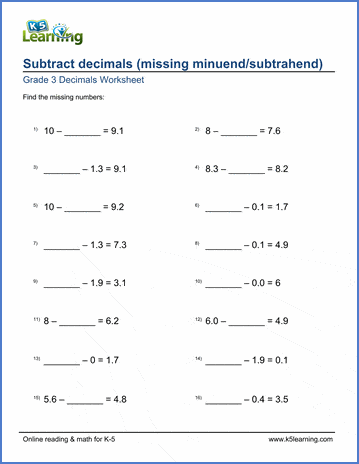# Subtract decimals (missing numbers)

## Worksheets:  subtracting decimals with missing minuends/subtrahends

Below are six versions of our grade 3 math worksheet on subtracting decimals with missing minuends or subtrahends. All numbers are either whole numbers or have one decimal digit. These worksheets are pdf files.## More fractions worksheets

Explore all of our fractions worksheets, from dividing shapes into "equal parts" to multiplying and dividing improper fractions and mixed numbers.

## What is K5?

K5 Learning offers reading and math worksheets, workbooks and an online reading and math program for kids in kindergarten to grade 5.  We help your children build good study habits and excel in school.